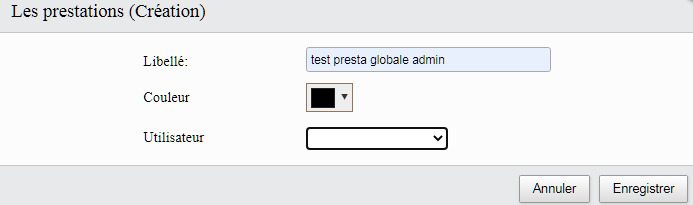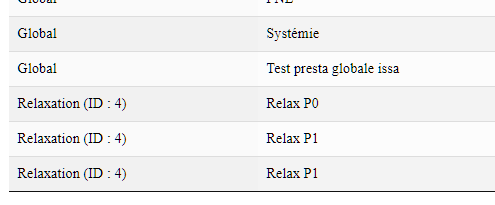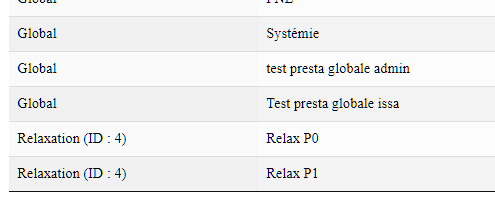# after create the datatable do not display created row while refresh browser

Hi all
Hope you are fine
I get a strange problem:
php

``````// \$IsAdmin, \$IsSA are boolean
// \$UsrID is long int

Editor::inst( \$db, \$TblName )
->fields(
Field::inst( 'prestations.ID'),
Field::inst( 'prestations.Libelle')
->validator( Validate::notEmpty( ValidateOptions::inst()
->message( 'le libellé ne peut être vide' )
)
),
Field::inst( 'prestations.Couleur' ),
Field::inst( 'prestations.ID_Utilisateur')
->options( extOptions::inst()
->pre(array(array('value'=>'','label'=>'')))
->table('utilisateurs')
->value('ID')
->label(array('Nom','Prenom'))
->render(function(\$row){
return \$row['Nom'].' '.\$row['Prenom'].' ('.\$row['ID'].')';
})
->where( function(\$q) use (\$IsSA, \$IsAdmin, \$UsrID) {
if( ! \$IsSA ) {
\$q->where('ID', \$UsrID, '=');
};
};
})
)
->setFormatter( 'Format::ifEmpty', null )
,
Field::inst( 'utilisateurs.Nom' )
)
->leftJoin( 'utilisateurs', 'utilisateurs.ID', '=', 'prestations.ID_Utilisateur' )
->where( function ( \$q ) use ( \$IsAdmin, \$IsSA, \$UsrID ) {
if( ! \$IsSA ) {
\$q->or_where('utilisateurs.ID', \$UsrID, '=');
\$q->or_where('utilisateurs.ID',null,'=');
}
};
})
->on( 'postCreate', function ( \$editor, \$id, \$values, \$row ) use( \$TblName)  {logChange( 'C', \$id, \$TblName, \$values );} )
->on( 'postEdit', function ( \$editor, \$id, \$values, \$row ) use( \$TblName)  {logChange( 'M', \$id, \$TblName, \$values );} )
->on( 'postRemove', function ( \$editor, \$id, \$values ) use( \$TblName)  {logChange( 'S', \$id, \$TblName, \$values );} )

->process( \$_POST )
->json();
``````

on create a row, the table does not display created record while I ask to refresh page...after create (duplicated row)After refreshyou can have a look at http://test.dafaction.appinfo.fr/ using user / pass as : NUJBD6DE / NUJBD6DE

Thanks

• Hi,

Thanks for the test case - I've added a few test rows you might want to delete!

It is returning JSON that is being added to the DataTable, but it is always returning this row when adding a new one:

``````{
"data": [{
"DT_RowId": "row_9",
"prestations": {
"ID": "9",
"Libelle": "Relax P1",
"Couleur": "#1a22ed",
"ID_Utilisateur": "4"
},
"utilisateurs": {
"Nom": "Relaxation"
}
}]
}
``````

Could you add `->debug(true)` immediately before the `->process()` call please in your PHP?

Also what is `\$TblName`, and is it's primary key an auto incrementing integer?

Allan

Hi @allan

Thanks

debug added in php, table structure below

table distance (that has more fields) use exactly same join and where conditions and goes normally.

I include distance sample...

php used for distance :

``````Editor::inst( \$db, \$TblName )
->fields(
Field::inst( 'distances.ID'),
Field::inst( 'distances.Libelle')
->validator( Validate::notEmpty( ValidateOptions::inst()
->message( 'le libellé ne peut être vide' )
)
),
Field::inst( 'distances.Couleur' ),
Field::inst( 'distances.Mini' ),
Field::inst( 'distances.Maxi' ),
Field::inst( 'distances.ID_Utilisateur')
->options( extOptions::inst()
->pre(array(array('value'=>'','label'=>'')))
->table('utilisateurs')
->value('ID')
->label(array('Nom','Prenom'))
->render(function(\$row){
return \$row['Nom'].' '.\$row['Prenom'].' ('.\$row['ID'].')';})
->where( function(\$q) use (\$IsSA, \$IsAdmin, \$UsrID) {
if( ! \$IsSA ) {
\$q->or_where('utilisateurs.ID', \$UsrID, '=');
};
})
)
->setFormatter( 'Format::ifEmpty', null )
Field::inst( 'utilisateurs.Nom' )
)
->leftJoin( 'utilisateurs', 'utilisateurs.ID', '=', 'distances.ID_Utilisateur' )
->where( function ( \$q ) use ( \$IsAdmin, \$IsSA, \$UsrID ) {
if( ! (\$IsSA || \$IsAdmin)) {\$q->where('distances.ID_Utilisateur', \$UsrID, '=');}
})
->on( 'postCreate', function ( \$editor, \$id, \$values, \$row ) use( \$TblName)  {logChange( 'C', \$id, \$TblName, \$values );} )
->on( 'postEdit', function ( \$editor, \$id, \$values, \$row ) use( \$TblName)  {logChange( 'M', \$id, \$TblName, \$values );} )
->on( 'postRemove', function ( \$editor, \$id, \$values ) use( \$TblName)  {logChange( 'S', \$id, \$TblName, \$values );} )

->process( \$_POST )
->json();
``````

table structure :

```
-- table distances
CREATE TABLE IF NOT EXISTS `distances` (
`ID` int(10) UNSIGNED NOT NULL AUTO_INCREMENT,
`ID_Utilisateur` bigint(20) UNSIGNED DEFAULT NULL,
`Libelle` varchar(50) DEFAULT NULL,
`Mini` int(11) DEFAULT NULL,
`Maxi` int(11) DEFAULT NULL,
`Couleur` varchar(10) DEFAULT NULL,
PRIMARY KEY (`ID`),
KEY `UsrDistance` (`ID_Utilisateur`)
) ENGINE=InnoDB AUTO_INCREMENT=6 DEFAULT CHARSET=utf8;

-- table prestations
CREATE TABLE IF NOT EXISTS `prestations` (
`ID` bigint(20) UNSIGNED NOT NULL AUTO_INCREMENT,
`ID_Utilisateur` bigint(20) UNSIGNED DEFAULT NULL,
`Libelle` varchar(30) NOT NULL,
`Couleur` varchar(10) DEFAULT NULL,
PRIMARY KEY (`ID`),
KEY `prestations_Utilisateur` (`ID_Utilisateur`),
KEY `prestations_Couleur` (`Couleur`)
) ENGINE=InnoDB DEFAULT CHARSET=utf8;

-- table utilisateurs
CREATE TABLE IF NOT EXISTS `utilisateurs` (
`ID` bigint(20) UNSIGNED NOT NULL AUTO_INCREMENT,
`ID_Civilites` int(10) UNSIGNED DEFAULT NULL,
`Nom` varchar(30) DEFAULT NULL,
`Prenom` varchar(30) DEFAULT NULL,
`Adresse` varchar(30) DEFAULT NULL,
`Adresse_Suite` varchar(30) DEFAULT NULL,
`Ville` varchar(50) DEFAULT NULL,
`CodePostal` varchar(5) DEFAULT NULL,
`ID_Departement` int(10) UNSIGNED DEFAULT NULL,
`ID_Commune` int(10) UNSIGNED DEFAULT NULL,
`Telephone` varchar(20) DEFAULT NULL,
`Email` varchar(50) DEFAULT NULL,
`ID_Statut` int(10) UNSIGNED DEFAULT NULL,
`Login` char(20) NOT NULL,
`Password` char(64) DEFAULT NULL,
`Actif` tinyint(1) NOT NULL DEFAULT '1',
`Administrateur` tinyint(1) NOT NULL DEFAULT '0',
`ID_Session` varchar(50) DEFAULT NULL,
`IP_Session` varchar(50) DEFAULT NULL,
`Visible` tinyint(1) NOT NULL DEFAULT '1',
`DateCreation` datetime DEFAULT CURRENT_TIMESTAMP,
`Siret` varchar(20) DEFAULT NULL,
`Sigle` varchar(50) DEFAULT NULL,
`LogoID` bigint(20) UNSIGNED DEFAULT NULL,
`TamponID` bigint(20) UNSIGNED DEFAULT NULL,
`Prefix` varchar(4) DEFAULT NULL,
`NumFac` int(3) DEFAULT NULL,
`Lon` float DEFAULT NULL,
`Lat` float DEFAULT NULL,
`ExoTVA` tinyint(1) DEFAULT '0',
PRIMARY KEY (`ID`),
KEY `ID_Ville` (`ID_Commune`),
KEY `ID_Civilites` (`ID_Civilites`),
KEY `ID_Departement` (`ID_Departement`),
KEY `ID_Statut` (`ID_Statut`) USING BTREE,
KEY `Logo` (`LogoID`),
KEY `Tampon` (`TamponID`)
) ENGINE=InnoDB AUTO_INCREMENT=6 DEFAULT CHARSET=utf8;

--

## -- Contraintes pour la table `prestations`

ALTER TABLE `prestations`
ADD CONSTRAINT `prestations_Utilisateur` FOREIGN KEY (`ID_Utilisateur`) REFERENCES `utilisateurs` (`ID`);

## -- Contraintes pour la table `distances`

ALTER TABLE `distances`
ADD CONSTRAINT `UsrDistance` FOREIGN KEY (`ID_Utilisateur`) REFERENCES `utilisateurs` (`ID`);

hi @allan

do you find why ?

• edited November 2020

Also what is \$TblName, and is it's primary key an auto incrementing integer?
Allan

I don't see ->debug(true) in your PHP code, and you have not explained \$TblName.

Hi

\$TblName is a switch value... in fact the table name.
In first sample, this is prestations, in second distances
I do add ->debug(true) in php code :

``````switch (\$TblName) {

case 'distances': {
Editor::inst( \$db, \$TblName )
->fields(
Field::inst( 'distances.ID'),
Field::inst( 'distances.Libelle')
->validator( Validate::notEmpty( ValidateOptions::inst()
->message( 'le libellé ne peut être vide' )
)
),
Field::inst( 'distances.Couleur' ),
Field::inst( 'distances.Mini' ),
Field::inst( 'distances.Maxi' ),
Field::inst( 'distances.ID_Utilisateur')
->options( extOptions::inst()
->pre(array(array('value'=>'','label'=>'')))
->table('utilisateurs')
->value('ID')
->label(array('Nom','Prenom'))
->render(function(\$row){
return \$row['Nom'].' '.\$row['Prenom'].' ('.\$row['ID'].')';
})
->where( function(\$q) use (\$IsSA, \$IsAdmin, \$UsrID) {
if( ! \$IsSA ) {
\$q->where('ID', \$UsrID, '=');
} else {
\$q->or_where('ID', \$UsrID, '=');
};
};
})
)
->setFormatter( 'Format::ifEmpty', null )
,
Field::inst( 'utilisateurs.Nom' )
)
->leftJoin( 'utilisateurs', 'utilisateurs.ID', '=', 'distances.ID_Utilisateur' )

->where( function ( \$q ) use ( \$IsAdmin, \$IsSA, \$UsrID ) {
if( ! (\$IsSA || \$IsAdmin)) {\$q->where('distances.ID_Utilisateur', \$UsrID, '=');}
})

->on( 'postCreate', function ( \$editor, \$id, \$values, \$row ) use( \$TblName)  {logChange( 'C', \$id, \$TblName, \$values );} )
->on( 'postEdit', function ( \$editor, \$id, \$values, \$row ) use( \$TblName)  {logChange( 'M', \$id, \$TblName, \$values );} )
->on( 'postRemove', function ( \$editor, \$id, \$values ) use( \$TblName)  {logChange( 'S', \$id, \$TblName, \$values );} )

->debug(true)
->process( \$_POST )
->json();
break;
}

case 'prestations': {
Editor::inst( \$db, \$TblName )
->fields(
Field::inst( 'prestations.ID'),
Field::inst( 'prestations.Libelle')
->validator( Validate::notEmpty( ValidateOptions::inst()
->message( 'le libellé ne peut être vide' )
)
),
Field::inst( 'prestations.Couleur' ),
Field::inst( 'prestations.ID_Utilisateur')
->options( extOptions::inst()
->pre(array(array('value'=>'','label'=>'')))
->table('utilisateurs')

->value('ID')
->label(array('Nom','Prenom'))
->render(function(\$row){
return \$row['Nom'].' '.\$row['Prenom'].' ('.\$row['ID'].')';
})
->where( function(\$q) use (\$IsSA, \$IsAdmin, \$UsrID) {
if( ! \$IsSA ) {
\$q->where('ID', \$UsrID, '=');
};
};
})
)
->setFormatter( 'Format::ifEmpty', null )
,
Field::inst( 'utilisateurs.Nom' )
)
->leftJoin( 'utilisateurs', 'utilisateurs.ID', '=', 'prestations.ID_Utilisateur' )
->where( function ( \$q ) use ( \$IsAdmin, \$IsSA, \$UsrID ) {
if( ! \$IsSA ) {
\$q->or_where('utilisateurs.ID', \$UsrID, '=');
\$q->or_where('utilisateurs.ID',null,'=');
}
};
})

->on( 'postCreate', function ( \$editor, \$id, \$values, \$row ) use( \$TblName)  {logChange( 'C', \$id, \$TblName, \$values );} )
->on( 'postEdit', function ( \$editor, \$id, \$values, \$row ) use( \$TblName)  {logChange( 'M', \$id, \$TblName, \$values );} )
->on( 'postRemove', function ( \$editor, \$id, \$values ) use( \$TblName)  {logChange( 'S', \$id, \$TblName, \$values );} )

->debug(true)
->process( \$_POST )
->json();
break;
}
}
....
``````
• Hi,

Thanks - I've just been digging into this and it is due to the OR condition in the SQL query:

``````WHERE `utilisateurs`.`Administrateur` = :where_0 OR `utilisateurs`.`ID` = :where_1 OR `utilisateurs`.`ID` IS NULL AND `prestations`.`id` = :where_3 "
``````

The query isn't strict enough (or perhaps "grouped enough" is the right term?).

I don't actually see any `or_where` lines in the code given above, so I assume that it isn't the complete code? You need to add a grouping around your OR conditions. See this example.

Allan

edited November 2020

Hi @allan

This is the complete code...
After refresh the where condition do not change, but row is correctly displayed...

There is not where clause about `prestations`.`id`.
I don't see it in this code not ?

``````WHERE `utilisateurs`.`Administrateur` = :where_0
OR `utilisateurs`.`ID` = :where_1
OR `utilisateurs`.`ID` IS NULL
// I don't find these condition in my code...
AND `prestations`.`id` = :where_3 "

``````

In fact I want to

```````utilisateurs`.`Administrateur` = :where_0
``````

show row for current user (if he is admin too)

``````OR `utilisateurs`.`ID` = :where_1
``````

and if current user is admin show row with no owner

``````// if (\$IsAdmin) {\$q->or_where('utilisateurs.ID',null,'=');}

OR `utilisateurs`.`ID` IS NULL
``````

do I miss something ?

Hi @allan

Sorry but your sample does not give me a response...

The expression

``````->where( function ( \$q ) use ( \$IsAdmin, \$IsSA, \$UsrID ) {
if( ! \$IsSA ) {
\$q->or_where('utilisateurs.ID', \$UsrID, '=');
\$q->or_where('utilisateurs.ID',null,'=');
}
};
})
``````

should return :

``````// if not \$IsAdmin
where 'utilisateurs.Administrateur' = 0 OR 'utilisateurs.ID' = \$UsrID

where 'utilisateurs.Administrateur' = 0 OR 'utilisateurs.ID' = \$UsrID OR 'utilisateurs.ID' ID null
``````

and this is what I expect

Other question.... if the where clause display row correctly on refresh, the same condition should show the same row after create, not ? Actually this return a duplicate row.... Do you mind a incorrect where clause should show duplicate row ?

Where am I wrong ?

Thanks
Bob

Hi
I do update the sample shown... The where clause is strictly the same for distances and prestations, but the result not...
Adding in distances is OK, in prestations NOK

``````    switch (\$TblName) {

case 'distances': {
Editor::inst( \$db, \$TblName )
->fields(
Field::inst( 'distances.ID'),
Field::inst( 'distances.Libelle')
->validator( Validate::notEmpty( ValidateOptions::inst()
->message( 'le libellé ne peut être vide' )
)
),
Field::inst( 'distances.Couleur' ),
Field::inst( 'distances.Mini' )
// ->validator( Validate::notEmpty(
// ValidateOptions::inst()
// ->message( 'merci de saisir la distance mini SVP' )
// )
->validator( Validate::minMaxNum( 0, 999, '.',
ValidateOptions::inst()
->message( 'la distance minimale doit être comprise entre 0 et 999' )
)
),
Field::inst( 'distances.Maxi' )
// ->validator( Validate::notEmpty(
// ValidateOptions::inst()
// ->message( 'merci de saisir la distance maxi SVP' )
// )
->validator(
Validate::minMaxNum( 1, 1000, '.',
ValidateOptions::inst()
->message( 'la distance maximale doit être comprise entre 1 et 1000' )
)
),
Field::inst( 'distances.ID_Utilisateur')
->options( extOptions::inst()
->pre(array(array('value'=>'','label'=>'')))
->table('utilisateurs')
->value('ID')
->label(array('Nom','Prenom'))
->render(function(\$row){
return \$row['Nom'].' '.\$row['Prenom'].' ('.\$row['ID'].')';
})
->where( function(\$q) use (\$IsSA, \$IsAdmin, \$UsrID) {
if( ! \$IsSA ) {
\$q->where('ID', \$UsrID, '=');
};
};
})
)
->setFormatter( 'Format::ifEmpty', null )
,
Field::inst( 'utilisateurs.Nom' )
)
->leftJoin( 'utilisateurs', 'utilisateurs.ID', '=', 'distances.ID_Utilisateur' )
->where( function ( \$q ) use ( \$IsAdmin, \$IsSA, \$UsrID ) {
if( ! \$IsSA ) {
\$q->where('utilisateurs.ID', \$UsrID, '=');
\$q->or_where('utilisateurs.ID',null,'=');
};
};
})
->on( 'postCreate', function ( \$editor, \$id, \$values, \$row ) use( \$TblName)  {logChange( 'C', \$id, \$TblName, \$values );} )
->on( 'postEdit', function ( \$editor, \$id, \$values, \$row ) use( \$TblName)  {logChange( 'M', \$id, \$TblName, \$values );} )
->on( 'postRemove', function ( \$editor, \$id, \$values ) use( \$TblName)  {logChange( 'S', \$id, \$TblName, \$values );} )
->process( \$_POST )
->json();
break;
}

case 'prestations': {
Editor::inst( \$db, \$TblName )
->fields(
Field::inst( 'prestations.ID'),
Field::inst( 'prestations.Libelle')
->validator( Validate::notEmpty( ValidateOptions::inst()
->message( 'le libellé ne peut être vide' )
)
),
Field::inst( 'prestations.Couleur' ),
Field::inst( 'prestations.ID_Utilisateur')
->options( extOptions::inst()
->pre(array(array('value'=>'','label'=>'')))
->table('utilisateurs')
->value('ID')
->label(array('Nom','Prenom'))
->render(function(\$row){
return \$row['Nom'].' '.\$row['Prenom'].' ('.\$row['ID'].')';
})
->where( function(\$q) use (\$IsSA, \$IsAdmin, \$UsrID) {
if( ! \$IsSA ) {
\$q->where('ID', \$UsrID, '=');
};
};
})
)
->setFormatter( 'Format::ifEmpty', null )
,
Field::inst( 'utilisateurs.Nom' )
)
->leftJoin( 'utilisateurs', 'utilisateurs.ID', '=', 'prestations.ID_Utilisateur' )
->where( function ( \$q ) use ( \$IsAdmin, \$IsSA, \$UsrID ) {
if( ! \$IsSA ) {
\$q->where('utilisateurs.ID', \$UsrID, '=');
\$q->or_where('utilisateurs.ID',null,'=');
};
};
})
->on( 'postCreate', function ( \$editor, \$id, \$values, \$row ) use( \$TblName)  {logChange( 'C', \$id, \$TblName, \$values );} )
->on( 'postEdit', function ( \$editor, \$id, \$values, \$row ) use( \$TblName)  {logChange( 'M', \$id, \$TblName, \$values );} )
->on( 'postRemove', function ( \$editor, \$id, \$values ) use( \$TblName)  {logChange( 'S', \$id, \$TblName, \$values );} )
->process( \$_POST )
->json();
break;
}
``````
• There is not where clause about prestations.id.

Editor adds it so it will only get the data required.

You need to wrap your `or_where` statements in another `where` function - e.g.:

``````->where( function ( \$q ) use ( \$IsAdmin, \$IsSA, \$UsrID ) {
if( ! \$IsSA ) {
\$q->where( function \$(r) use ( \$IsAdmin, \$IsSA, \$UsrID ) {
\$r->where('utilisateurs.ID', \$UsrID, '=');

\$r->or_where('utilisateurs.ID',null,'=');
};
});
};
})
``````

That inner `where` will automatically group your `X AND Y OR Z` in parenthesis for SQL.

Allan

Hi Allan
Thanks for this response.
I did not know editor was adding a where condition...
So I'll do as you tell me

But, can you explain why after refresh this where sentence does what I expect (just for information)

Best regards
Bob

edited November 2020

and why with distance this where clause run OK ?

The condition being run before was:

``````WHERE
`utilisateurs`.`ID` = :where_1 OR
`utilisateurs`.`ID` IS NULL AND
`prestations`.`id` = :where_3 "
``````

Note the lack of parenthesis. That is the same as `A or B or (C and D)`.

You want:

``````WHERE
(
`utilisateurs`.`ID` = :where_1 OR
`utilisateurs`.`ID` IS NULL
) AND
`prestations`.`id` = :where_3 "
``````

which that inner `->where()` will do. Does for that method available here.

Allan

Hi @allan
Sorry for that but I can't understant why after create and after refresh this (wrong) filter should produce different result...
I apologies for this question but if you could explain this point...

After the create action it added ``prestations`.`id`= :where_3 "` so that it will only read back the newly created row.

Allan

Thank Allan

Do you think a global parenthesis on where clause passed should be added before adding the inner where, so result should be the same before and after refresh ?

Bobby